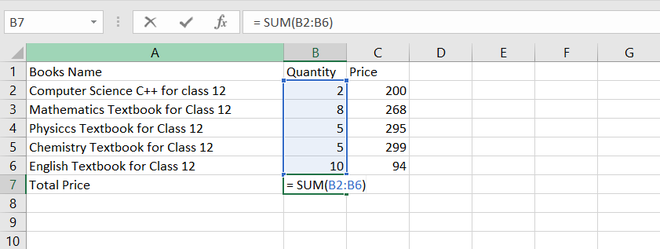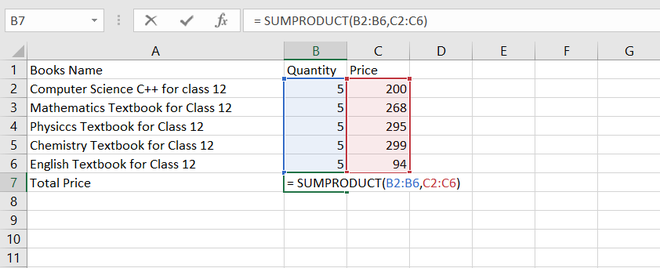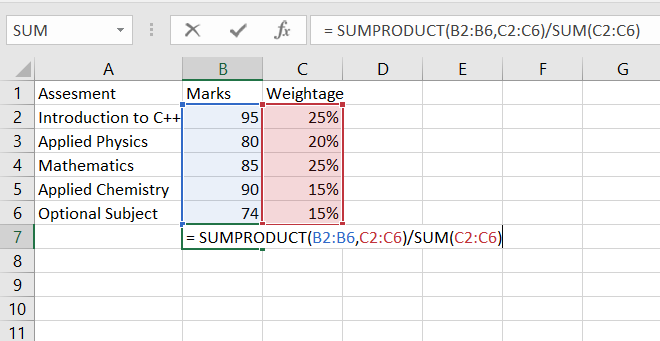# How to Calculate Weighted Average in Excel?

• Last Updated : 15 May, 2021

Have you ever think why we need an average or weightage average? In our daily, we can’t calculate everything, let’s suppose I am asking how’s the weather for a week, you are not going to tell me every day’s temperature, but an estimation here comes to the concept of an Average. Mathematically you can evaluate the average=  Sum of Values/Number of Values, and the values carry equal weightage.

Now let’s take another example you are in university, and you got your result of the semester exam. Since the semester consists of minor and major where minor carries 25% weightage and major carries 75% of weightage, now how will you calculate your average? So here comes a concept of weightage average where we consider the weight of every value.

## How To Calculate Weighted Average in Excel?

In order to calculate the weighted average, we will use two functions in Excel, the first one is the sum function, and the other SUMPRODUCT function, let’s discuss it one by one.

### SUM Function

It is an inbuilt function in Excel, helps to sum the numerical data in a range of cells. The function enables us to calculate multiple data very easily.

`= SUM (number1, [number2],... )`

For example, you are purchasing class 12  science textbooks for donating to the NGO, so now you have to calculate the total number of books, so here you simply use the sum function.### SUMPRODUCT Function

It is an inbuilt function in Excel, it will return the sum of products of the value given in the argument or an array. This function usually uses when you need to multiply many cells together.

`= SUMPRODUCT ( array1, [array2],[array3],...)`

For example, you are purchasing class 12 textbooks for donating to the NGO, so now you have to calculate the total price.  So here’s this function comes handy, you will first enter array 1 and the put comma and again array 2 and then press enter, you will get your result, which is 5780.Now we are clear with the concept of both SUM function and SUMPRODUCT function now let’s calculate the weightage average. So in Excel to calculate weightage average is SUMPRODUCT/ SUM.

`Weightage Average = SUMPRODUCT ( array1, [array2],[array3],...)/SUM (number1, [number2],...) `

Let’s understand with an example, you have to calculate your college marks, but every subject has a different average. So we can use the weightage average to calculate our average marks.  So Now put the values in the formula which is:

` weightage average = SUMPRODUCT(B1:B6, C2: C6)/SUM(C2:C6)`

And then hit enter, you will get your result 82.25.Example of weightage average

My Personal Notes arrow_drop_up